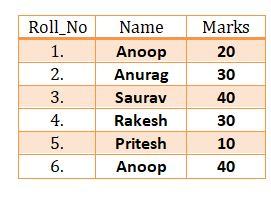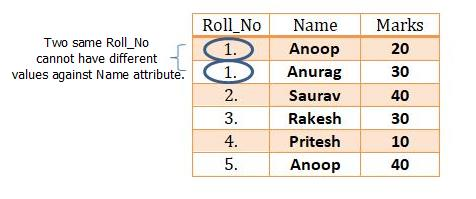# FUNCTIONAL DEPENDENCY IN DBMS

Functional Dependency In DBMS(FDs) | DBMS Tutorial | Minigranth

# Functional Dependency In DBMS : Introduction

• Functional Dependency in DBMS also known as “FDs” means a relationship. A relationship which only exists when an attribute can determine other attribute functionally.
• The first attribute does not computes or calculates the value of second attribute but searches value of the tuple corresponding to the first attribute and fetches that value of the attribute.
• Functional Dependency in DBMS is denoted using an arrow between two or more attributes such as :

FD : A B

Here, A & B are the attributes present in any relation.

• B” means, “B” is functionally dependent upon “A” or “A” functionally determines “B”. Functional dependency acts as a constraint between set of attributes present in any database.

### Functional Dependency In DBMS : Examples

• Functional Dependency in DBMS and Keys are the most important concepts that are used as a foundation in database normalization. We will try to explain you with example how actually functional dependency works.

Example-1 : Consider a table student_details containing details of some students.Example : student_details Table

We can conclude from Roll_No attribute in the table, we are able to determine the Name of student uniquely and same is the case with marks too. Hence, we can say that Name and Marks are functionally dependent on Roll_No but the vice versa is not true.

FD1 : Roll_No   Name

FD2 : Roll_No   Marks

 NOTE : In the above scenario, Two Roll_No can have same Name(i.e. Anoop in the above table as Roll_No-1 and Roll_No-5 but two same Name cannot have same Roll_No. This is how Functional dependency in DBMS concept works.

Example-2 : Consider the table student_details containing details of some students.Example : student_details Table

• Here, Name is not functionally dependent upon Roll_No as whenever we will try to search the value of Name against Roll_No attribute, two different names will be provided which is practically not possible.
• Hence, Functional Dependency in DBMS concept exists when an attribute is able to uniquely determine another attribute.

### Functional Dependency In DBMS : Armstrong’s Axioms

• Axioms in database management systems was introduced by William W. Armstrong in late 90’s and these axioms play a vital role while implementing the concept of functional dependency in DBMS for database normalization. There exists six inferences known a s “Armstrong’s Axioms” which are discussed below.
1. Reflexive : It means, if set “B” is a subset of “A”, then A  B.
2. Augmentation : It means, if A  B, then AC  BC.
3. Transitive : It means, if A  B & B  C, then A  C.
4. Decomposition : It means, if A  BC, then A  B & A  C.
5. Union : It means, if A  B & A  C, then A  BC.
6. Pseudo-Transitivity : It means, if A  B and DB  C, then DA  C.
 NOTE : These rules are helpful while dealing with determination of closure of functional dependency in DBMS and calculation of canonical cover. Both of these topics are discussed in the next chapter.